# GMAT Math : Understanding measurement

## Example Questions

← Previous 1 3

### Example Question #1 : Understanding MeasurementNote: Figure NOT drawn to scale.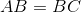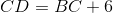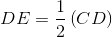Give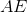in terms of.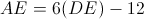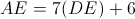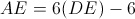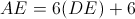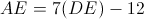Explanation:

Since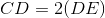Since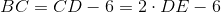, and, subsequently,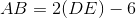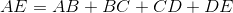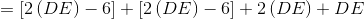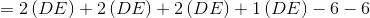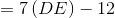### Example Question #1 : Measurement Problems

Big Bob the, 8-feet tall warehouse foreman is stacking uniformly sized boxes of goods in the warehouse. Big Bob notices that he is the exact same height as 3 boxes stacked on top of each other. If the warehouse ceiling is 55 feet high, what is the maximum number of boxes that he can stack on top of one another before he hits the ceiling?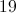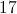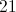Explanation:

To find the height of each box set the 3 boxes equal to the height of Big Bob. So, letbe the height of each box. Then,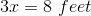or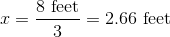Therefore to find the maximum number of boxes that fit under the ceiling, we divide the ceiling height by the height of each box. So,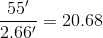or 20 whole boxes would fit under the ceiling.

### Example Question #3 : Understanding Measurement

If the length and width of a rectangular room is increased by 20%, what percent has the area increased by?Not sufficient information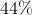Explanation: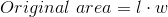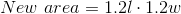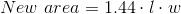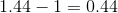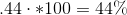### Example Question #4 : Understanding Measurement

Two bedrooms have the same area. Bedroom A is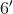by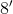and the width of Bedroom B is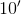. Find the length of Bedroom B.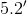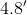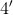Explanation:

Area of Bedroom A is equal to area of Bedroom B, therefore: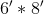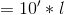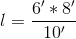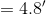### Example Question #5 : Understanding Measurement

Restate 200 feet per second in miles per hour (nearest whole number).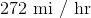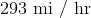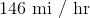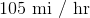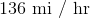Explanation:

One mile is equal to 5,280 feet, and one hour is equal to 3,600 seconds, The speed can be converted as follows: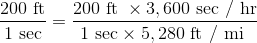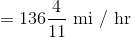or, rounded, 136 miles per hour.

### Example Question #6 : Understanding Measurement

A unit of distance used in marine navigation is the nautical mile; what we call a mile on land is also referred to as a statute mile.

One nautical mile is defined as 1,852 meters. Using the conversion factor 1.609 kilometers = 1 statute mile, how many statute miles are equal to 1,000 nautical miles (nearest whole number)?

1,151 statute miles

869 statute miles

2,980 statute miles

1,609 statute miles

622 statute miles

1,151 statute miles

Explanation:

1,000 nautical miles are equal to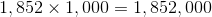meters, or

1 statute mile is equal to 1.609 kilometers, or 1,609 meters; divide 1,852,000 meters by 1,609 meters per statute miles to get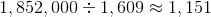statute miles, the equivalent of 1,000 nautical miles.

### Example Question #7 : Understanding Measurement

A furlong is a unit of length used in horse racing; it is equal to one-eighth of a mile. To the nearest tenth, how many meters are equal to a furlong if 1.609 kilometers are equal to a mile?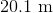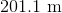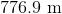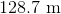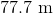Explanation:

1.609 kilometers, or 1,609 meters, are equal to a mile. One furlong, or one eighth of a mile, is equal to one eighth of 1,609 meters, or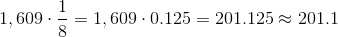meters.

### Example Question #8 : Understanding Measurement

A baseball travels at a rate of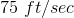.  How long does it take to reach a fence that is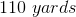away?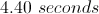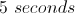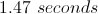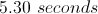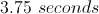Explanation:

The first step is to convert the distance into feet: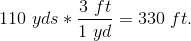Then, divide the distance by the baseball's rate of travel: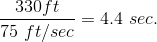### Example Question #9 : Understanding Measurement

It takes Jim 30 minutes to drive to work and 45 minutes to drive home from work.  What is Jim's average driving speed in miles per hour if Jim lives 25 miles from work?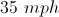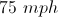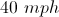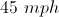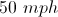Explanation:

The formula to find the average speed is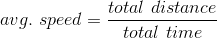.

Since the question deals with miles per hour, the time must be converted to hours from minutes: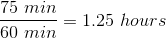Plugging the numbers into the equation gives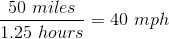.

### Example Question #10 : Understanding Measurement

Restate 100 miles per hour in feet per second (rounded to the nearest whole number).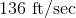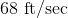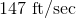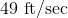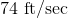Explanation:

One mile is equal to 5,280 feet, and one hour is equal to 3,600 seconds, The speed can be converted as follows: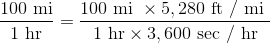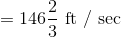or, rounded, 147 feet per second.

← Previous 1 3

Tired of practice problems?

Try live online GMAT prep today.